Adding real numbersPage 1

WATCH ALL SLIDES

Slide 1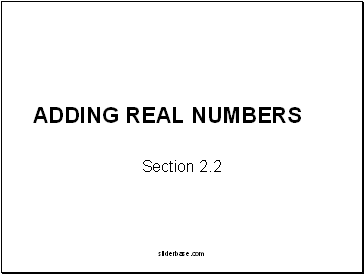sliderbase.com

Slide 2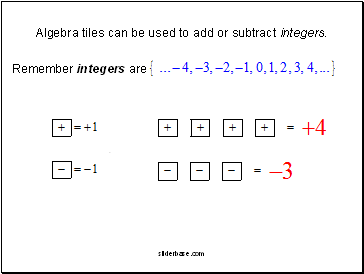Algebra tiles can be used to add or subtract integers.

Remember integers are

.

sliderbase.com

Slide 3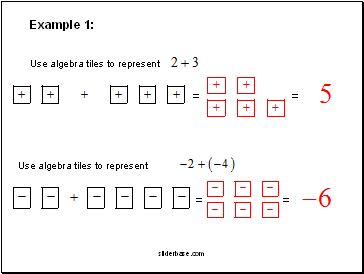Example 1:

Use algebra tiles to represent

=

=

Use algebra tiles to represent

=

=

sliderbase.com

Slide 4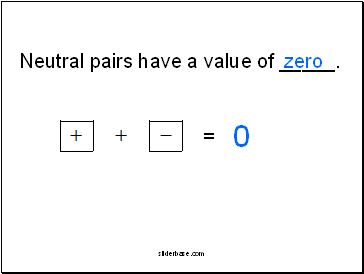Neutral pairs have a value of _.

zero

=

0

sliderbase.com

Slide 5Example 2:

A. Use algebra tiles to represent

and then cross out any neutral pairs.

+

= =

sliderbase.com

Slide 6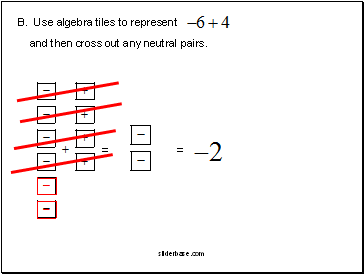B. Use algebra tiles to represent

and then cross out any neutral pairs.

=

=

sliderbase.com

Slide 7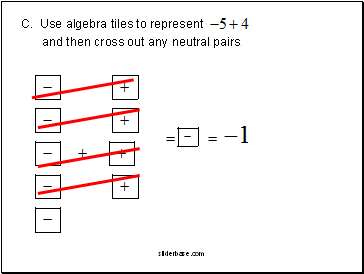C. Use algebra tiles to represent

and then cross out any neutral pairs.

sliderbase.com

Slide 8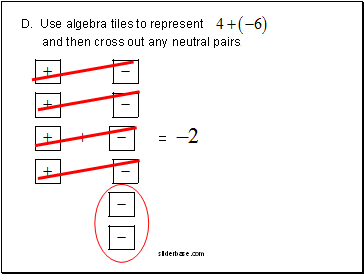D. Use algebra tiles to represent

and then cross out any neutral pairs.

=

sliderbase.com

Slide 9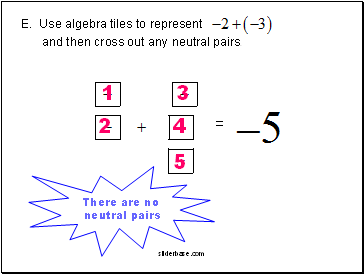E. Use algebra tiles to represent

and then cross out any neutral pairs.

There are no

neutral pairs

=

1

2

3

4

5

sliderbase.com

Slide 10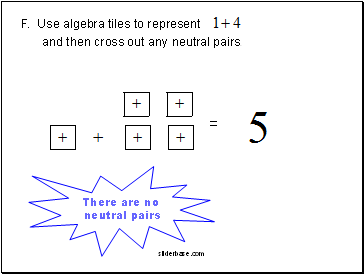F. Use algebra tiles to represent

and then cross out any neutral pairs.

There are no

neutral pairs

=

sliderbase.com

Slide 11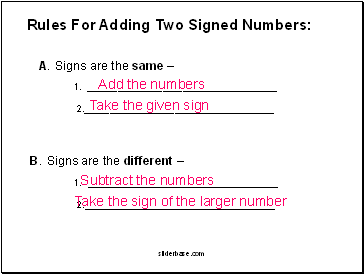Rules For Adding Two Signed Numbers:

A. Signs are the same –

2.

Take the given sign

B. Signs are the different –

2.

Subtract the numbers

Take the sign of the larger number

sliderbase.com

Slide 12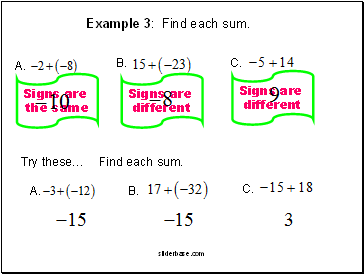Example 3: Find each sum.

A.

B.

C.

Signs are

the same

Signs are

different

Signs are

different

Try these… Find each sum.

A.

B.

C.

sliderbase.com

Slide 13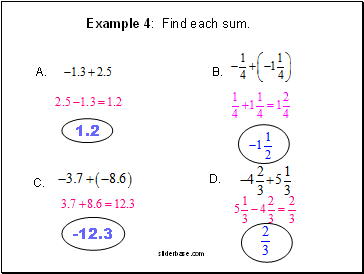Example 4: Find each sum.

A.

B.

C.

D.

1.2

-12.3

sliderbase.com

Go to page:
1  2